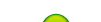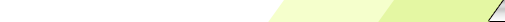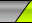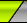Martes, 2023-10-03My siteMain | Registration | LoginWelcome Visitante | RSS
 Statistics Total en línea: 1 Invitados: 1 Usuarios: 0

# Anticovidian v.2 COVID-19: Hypothesis of the Lab Origin Versus a Zoonotic Event which can also be of a Lab Origin: https://zenodo.org/record/3988139

To go to Einstein 4: http://fdocc.ucoz.com/index/0-89

# Einstein pursues his reasoning:

§ 1. Defects of the Present View

As I have shown in the previous paper, the general theory of relativity requires that the universe be spatially finite. But this view of the universe necessitated an extension of equations (1), with the introduction of a new universal constant l, standing in a fixed relation to the total mass of the universe (or, respectively, to the equilibrium density of matter). This is gravely detrimental to the formal beauty of the theory [(1) is: Gmn  = – ½gmn G = - kTmn ]

§ 2. The Field Equations Freed of Scalars

The difficulties set forth above are removed by setting in place of field equations (1) the field equations

Gmn  = – ¼gmn G = - kTmn    ... (1a)

… We now write the field equations (1a) in the form

(Gmn  – ½gmn G) + ¼gmn G0 = - k [Tmn  + (1/4k)gmn (G - G0)] ... (9)

On the other hand, we transform the equations supplied with the cosmological term as already given

Gmn  lgmn  = - k (Tmn - ½gmn T).

Subtracting the scalar equation multiplied by ½, we next obtain

(Gmn  – ½gmn G) + gmn l = - kTmn

Now in regions where only electrical and gravitational fields are present, the right-hand side of this equation vanishes. For such regions we obtain, by forming the scalar,

- G + 4l = 0.

In such regions, therefore, the scalar of curvature is consistent, so that l may be replaced by ¼G0. Thus we may write the earlier field equation (1) in the form

Gmn  – ½gmn G + ¼gmn G0 = - kTmn  ... (10)

Comparing (9) with (10), we see that there is no difference between the new field equations and the earlier ones, except that instead of Tmn as tensor of "gravitational mass” there now occurs Tmn  + (1/4k)gmn (G - G0) which is independent of the scalar of curvature. But the new formulation has this great advantage, that the quantity l appears in the fundamental equations as a constant of integration, and no longer as a universal constant peculiar to the fundamental law.

§ 3. On the Cosmological Question

The last result already permits the surmise that with our new formulation the universe may be regarded as spatially finite, without any necessity for an additional hypothesis. As in the preceding paper I shall again show that with a uniform distribution of matter, a spherical world is compatible with the equations.

In the first place we set

ds2 = - gikdxidxk + dx42 (i, k = 1, 2, 3) … (11)

Then if Pik and P are, respectively, the curvature tensor of the second rank and the curvature scalar in the three-dimensional space, we have

Gik - ½gik G = Pik (i, k = 1, 2, 3)

Gi4 = G4i = G44 = 0

G = - P

- g = g.

It therefore follows for our case that

Gik - ½gik G = Pik - ½g ik P (i, k = 1, 2, 3)

G44 - ½g44G = ½P.

We pursue our reflexions, from this point on, in two ways. Firstly, with the support of equation (1a). Here Tmn denotes the energy-tensor of the electro-magnetic field, arising from the electrical particles constituting matter… our fundamental equations permit the idea of a spherical universe it is known (Cf. H. Weyl, "Raum, Zeit, Materie,” § 33) that this system is satisfied by a (three-dimensional) spherical universe

§ 4. Concluding Remarks

The above reflexions show the possibility of a theoretical construction of matter out of gravitational fiend and electro-magnetic field alone, without the introduction of hypothetical supplementary terms on the lines of Mie’s theory. This possibility appears particularly promising in that it frees us from the necessity of introducing a special constant l for the solution of the cosmological problem. On the other hand, there is a peculiar difficulty. For, if we specialize (1) for the spherically symmetrical static case we obtain one equation too few for defining the gmn  and fmn , with the result that any spherically symmetrical distribution of electricity appears capable of remaining in equilibrium. Thus the problem of the constitution of the elementary quanta cannot yet be solved on the immediate basis of the given field equations.

---------------------------------

Albert Einstein: Relativity

Relativity: The Special and General Theory © 1920, Publisher: Methuen & Co Ltd. First Published: December, 1916. Translated: Robert W. Lawson (Authorized translation).

Part III: Considerations on the Universe as a Whole

The Structure of Space According to the General Theory of Relativity

According to the general theory of relativity, the geometrical properties of space are not independent, but they are determined by matter… If we are to have in the universe an average density of matter which differs from zero, however small may be that difference, then the universe cannot be quasi-Euclidean. On the contrary, the results of calculation indicate that if matter be distributed uniformly, the universe would necessarily be spherical (or elliptical). Since in reality the detailed distribution of matter is not uniform, the real universe will deviate in individual parts from the spherical, i.e. the universe will be quasi-spherical. But it will be necessarily finite. In fact, the theory supplies us with a simple connection 1) between the space-expanse of the universe and the average density of matter in it.

#### 1)For the radius R of the universe we obtain the equation

R2 = (2/kp)

The use of the C.G.S. system in this equation gives 2/k = 1.08.1027; p is the average density of the matter and k is a constant connected with the Newtonian constant of gravitation.

Appendix IV

The Structure of Space According to the General Theory of Relativity

(Supplementary to Section 32)

Since the publication of the first edition of this little book, our knowledge about the structure of space in the large ("cosmological problem") has had an important development, which ought to be mentioned even in a popular presentation of the subject.

My original considerations on the subject were based on two hypotheses:

(1) There exists an average density of matter in the whole of space which is everywhere the same and different from zero.

(2) The magnitude ("radius") of space is independent of time.

Both these hypotheses proved to be consistent, according to the general theory of relativity, but only after a hypothetical term was added to the field equations, a term which was not required by the theory as such nor did it seem natural from a theoretical point of view ("cosmological term of the field equations").

Hypothesis (2) appeared unavoidable to me at the time, since I thought that one would get into bottomless speculations if one departed from it.

However, already in the 'twenties, the Russian mathematician Friedman showed that a different hypothesis was natural from a purely theoretical point of view. He realized that it was possible to preserve hypothesis (1) without introducing the less natural cosmological term into the field equations of gravitation, if one was ready to drop hypothesis (2). Namely, the original field equations admit a solution in which the "world radius" depends on time (expanding space). In that sense one can say, according to Friedman, that the theory demands an expansion of space.

A few years later Hubble showed, by a special investigation of the extra-galactic nebulae ("milky ways"), that the spectral lines emitted showed a red shift which increased regularly with the distance of the nebulae. This can be interpreted in regard to our present knowledge only in the sense of Doppler's principle, as an expansive motion of the system of stars in the large — as required, according to Friedman, by the field equations of gravitation. Hubble's discovery can, therefore, be considered to some extent as a confirmation of the theory.

There does arise, however, a strange difficulty. The interpretation of the galactic line-shift discovered by Hubble as an expansion (which can hardly be doubted from a theoretical point of view), leads to an origin of this expansion which lies "only" about 109 years ago [see below], while physical astronomy makes it appear likely that the development of individual stars and systems of stars takes considerably longer. It is in no way known how this incongruity is to be overcome.

I further want to remark that the theory of expanding space, together with the empirical data of astronomy, permit no decision to be reached about the finite or infinite character of (three-dimensional) space, while the original "static" hypothesis of space yielded the closure (finiteness) of space.

To go to Einstein 6: http://fdocc.ucoz.com/index/0-91

 Search
 Site friends Create your own site